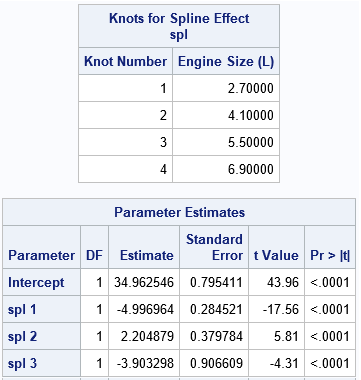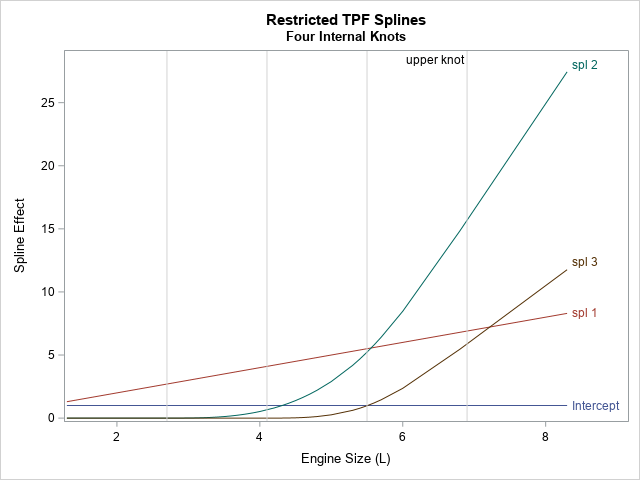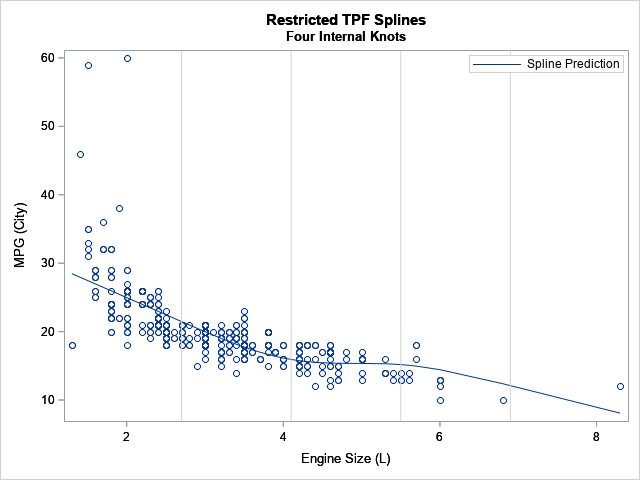The EFFECT statement is supported by more than a dozen SAS/STAT regression procedures. Among other things, it enables you to generate spline effects that you can use to fit nonlinear relationships in data. Recently there was a discussion on the SAS Support Communities about how to interpret the parameter estimates of spline effects. This article answers that question by visualizing the spline effects.

### An overview of generated effects

Spline effects are powerful because they enable you to use parametric models to fit nonlinear relationships between an independent variable and a response. Using spline effects is not much different than use polynomial effects to fit nonlinear relationships. Suppose that a response variable, Y, appears to depend on an explanatory variable, X, in a complicated nonlinear fashion. If the relationship looks quadratic or cubic, you might try to capture the relationship by introducing polynomial effects. Instead of trying to model Y by X, you might try to use X, X2, and X3.

Strictly speaking, polynomial effects do not need to be centered at the origin. You could translate the polynomial by some amount, k, and use shifted polynomial effects such as (X-k), (X-k)2, and (X-k)3. Or you could combine these shifted polynomials with polynomials at the origin. Or use shifted polynomials that are shifted by different amounts, such as by the constants k1, k2, and k3.

Spline effects are similar to (shifted) polynomial effects. The constants (such as k1, k2, k3) that are used to shift the polynomials are called knots. Knots that are within the range of the data are called interior knots. Knots that are outside the range of the data are called exterior knots or boundary knots. You can read about the various kinds of spline effects that are supported by the EFFECT statement in SAS. Rather than rehash the mathematics, this article shows how you can use SAS to visualize a regression that uses splines. The visualization clarifies the meaning of the parameter estimates for the spline effects.

### Output and visualize spline effects

This section shows how to output the spline effects into a SAS data set and plot the spline effects. Suppose you want to predict the MPG_City variable (miles per gallon in the city) based on the engine size. Because we will be plotting curves, the following statements sort the data by the EngineSize variable. Then the OUTDESIGN= option on the PROC GLMSELECT statement writes the spline effects to the Splines data set. For this example, I am using restricted cubic splines and four evenly spaced internal knots, but the same ideas apply to any choice of spline effects.

```/* Fit data by using restricted cubic splines. The EFFECT statement is supported by many procedures: GLIMMIX, GLMSELECT, LOGISTIC, PHREG, ... */ title "Restricted TPF Splines"; title2 "Four Internal Knots"; proc glmselect data=cars outdesign(addinputvars fullmodel)=Splines; /* data set contains spline effects */ effect spl = spline(EngineSize / details /* define spline effects */ naturalcubic basis=tpf(noint) /* natural cubic splines, omit constant effect */ knotmethod=equal(4)); /* 4 evenly spaced interior knots */ model mpg_city = spl / selection=none; /* fit model by using spline effects */ ods select ParameterEstimates SplineKnots; ods output ParameterEstimates=PE; quit;```The SplineKnots table shows the locations of the internal knots. There are four equally spaced knots because the procedure used the KNOTMETHOD=EQUAL(4) option. The ParameterEstimates table shows estimates for the regression coefficients for the spline effects, which are named "Spl 1", "Spl 2", and so forth. In the Splines data set, the corresponding variables are named Spl_1, Spl_2, and so forth.

But what do these spline effects look like? The following statements plot the spline effects versus the EngineSize variable, which is the variable from which the effects are generated:

```proc sgplot data=Splines; series x=EngineSize y=Intercept / curvelabel; series x=EngineSize y=spl_1 / curvelabel; series x=EngineSize y=spl_2 / curvelabel; series x=EngineSize y=spl_3 / curvelabel; refline 2.7 4.1 5.5 / axis=x lineattrs=(color="lightgray"); refline 6.9 / axis=x label="upper knot" labelloc=inside lineattrs=(color="lightgray"); yaxis label="Spline Effect"; run;```As stated in the documentation for the NATURALCUBIC option, these spline effects include "an intercept, the polynomial X, and n – 2 functions that are all linear beyond the largest knot," where n is the number of knots. This example uses n=4 knots, so Spl_2 and Spl_3 are the cubic splines. You will also see different spline effects if you change to one of the other supported spline methods, such as B-splines or the truncated power functions. Try it!

The graph shows that the natural cubic splines are reminiscent of polynomial effects, but there are a few differences:

• The spline effects (spl_2 and spl_3) are shifted away from the origin. The spl_2 effect is shifted by 2.7 units, which is the location of the first internal knot. The spl_3 effect is shifted by 4.1 units, which is the location of the second internal knot.
• The spline effects are 0 when EngineSize is less than the first knot position (2.7). Not all splines look like this, but these effects are based on truncated power functions (the TPF option).
• The spline effects are linear when EngineSize is greater than the last knot position (6.9). Not all splines look like this, but these effects are restricted splines.

### Predicted values are linear combinations of the spline effects

Visualizing the shapes of the spline effects enable you to make sense of the ParameterEstimates table. As in all linear regression, the predicted value is a linear combination of the design variables. In this case, the predicted values are formed by
Pred = 34.96 – 5*Spl_1 + 2.2*Spl_2 – 3.9*Spl_3
You can use the SAS DATA set or PROC IML to compute that linear combination of the spline effects. The following graph shows the predicted curve and overlays the locations of the knots:The coefficient for Spl_1 is the largest effect (after the intercept). In the graph, you can see the general trend has an approximate slope of -5. The coefficient for the Spl_2 effect is 2.2, and you can see that the predicted values change slope between the second and third knots due to adding the Spl_2 effect. Without the Spl_3 effect, the predicted values would continue to rise after the third knot, but by adding in a negative multiple of Spl_3, the predicted values turn down again after the third knot.

Notice that the prediction function for the restricted cubic spline regression is linear before the first knot and after the last knot. The prediction function models nonlinear relationships between the interior knots.

### Summary

In summary, the EFFECT statement in SAS regression procedures can generate spline effects for a continuous explanatory variable. The EFFECT statement supports many different types of splines. This article gives an example of using natural cubic splines (also called restricted cubic splines), which are based on the truncated power function (TPF) splines of degree 3. By outputting the spline effects to a data set and graphing them, you can get a better understanding of the meaning of the estimates of the regression coefficients. The predicted values are a linear combination of the spline effects, so the magnitude and sign of the regression coefficients indicate how the spline effects combine to predict the response.

The post Visualize a regression with splines appeared first on The DO Loop.